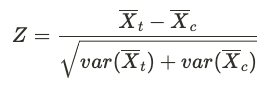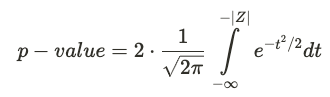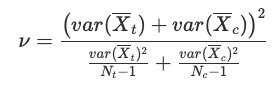# p-Value Calculation

In hypothesis testing, the p-value is the probability of observing an effect larger than or equal to the measured metric delta, under the assumption that the null hypothesis is true. In practice, a p-value that's lower than your pre-defined threshold is treated as evidence for there being a true effect.

The methodology used for p-value calculation depends on the number of degrees of freedom (ν). A two-sample z-test is appropriate for most experiments. Welch's t-test is used for smaller experiments with ν < 100. In both cases, the p-value depends on the metric mean and variance computed for the test and control groups.

### Two-Sample Z-Test​

The z-statistic of a two-sample z-test is:The two-sided p-value is obtained from the standard normal cumulative distribution function:### Welch's t-test​

For smaller sample sizes, Welch's t-test is the preferred statistical test for lower false positive rates in cases of unequal sizes and variances. In Pulse, Welch's t-test is automatically applied when the degress of freedom ν < 100.

The t-statistic is computed in the same way as the two-sample z-statistic above. Additionally, we compute the degrees of freedom ν using:The p-value is then obtained from the t-distribution with ν degrees of freedom.